# Spectrical Series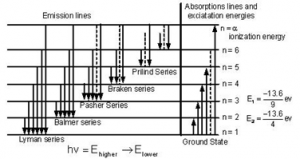When electrons moves around the nucleus in certain permitted orbit, it can jump from higher to the lower energy level with the emission of radiation. The wavelength of emitted radiation can be obtained by the expression: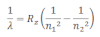Where R2= Rydberg constant
n1= lower energy level
n2 = higher energy level

On the basis of transition of electron from higher to lower energy level different spectral series can be obtained which are explained below

Lyman series:
If the transition of electron is to n1= 1 from n2 = 2,3,4…. Lyman series can be obtained. The wavelength of the layman series can be obtained as: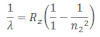he wavelength lies in the region of the ultraviolet.

Balmer series:
If the transition of electron is to n1=2 from n2 = 3,4,5… balmer series is obtained. The wave length of balmer series can be obtained as: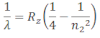The wavelength lies in the visible region.

Paschen series:
If the transition of electrons is to n1 = 3 from n2 = 3,5,6 …. paschen series can be obtained. The wavelength of paschen series is given by: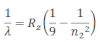he wavelength lies int eh infrared region.

Brackett series:
If the transition of electron is to n1= 4 from n2 = 5, 6 ,7 …. brakett series is obtaine . The wavelength of brackett series is given by: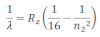The wavelength lies in the infrared region

Pfund series:
If the transition of electrons is to n1 = 5 from n2 = 6 ,7,8 … pfund series is obtained . The wavelength of pfund series is given by: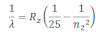The wavelength lies in the infrared region.